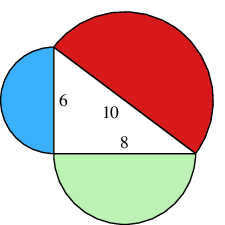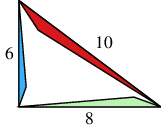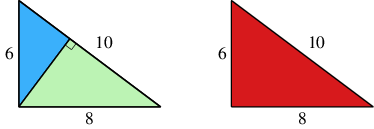#### You may also likeThis comes in two parts, with the first being less fiendish than the second. Itâ€™s great for practising both quadratics and laws of indices, and you can get a lot from making sure that you find all the solutions. For a real challenge (requiring a bit more knowledge), you could consider finding the complex solutions.### Discriminating

You're invited to decide whether statements about the number of solutions of a quadratic equation are always, sometimes or never true.This will encourage you to think about whether all quadratics can be factorised and to develop a better understanding of the effect that changing the coefficients has on the factorised form.

# The Quintessential Proof

##### Age 16 to 18Challenge LevelThis resource is from Underground Mathematics.

These first three questions are "warm-ups", designed to introduce the idea.  You are welcome to make use of Pythagoras's Theorem as you answer them.

In the following questions, the right-angled triangle has the specific side lengths $6$, $8$ and $10$.  Would your answers still be correct if we replaced them with other side lengths, say $a$, $b$ and $c$?

1. In the following figure, the red, blue and green regions are all semicircles.  How are their areas related?2. In the following figure, the red, blue and green regions are all equilateral triangles.  How are their areas related?3. In the following figure, the red, blue and green regions are all similar triangles. How are their areas related?#### The proof of Pythagoras's Theorem

In this final question, you are asked to use the ideas you have learnt in the earlier questions to prove Pythagoras's Theorem.  You may not assume Pythagoras's Theorem to be true when you answer this one!

In the left figure below, the blue and green regions have been formed by dropping a perpendicular as shown.  How are their areas related to the red area in the right figure?How does this result prove Pythagoras's Theorem?

This is an Underground Mathematics resource.

Underground Mathematics is hosted by Cambridge Mathematics. The project was originally funded by a grant from the UK Department for Education to provide free web-based resources that support the teaching and learning of post-16 mathematics.

Visit the site at undergroundmathematics.org to find more resources, which also offer suggestions, solutions and teacher notes to help with their use in the classroom.